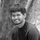cancel
Showing results for
Did you mean:

Fabric is Generally Available. Browse Fabric Presentations. Work towards your Fabric certification with the Cloud Skills Challenge.Microsoft

## Create calculated column based on text and probability%

Trying to create a calculated column to show the expected/probable revenue impact from a pipeline.

This means the column should do a function as:

If Probability = "10% - StageA" then 0.1*"Estimated revenue",

If Probability = "30% - StageB" then 0.3*"Estimated revenue",

If Probability = "95% - StageC" then 0.95*"Estimated revenue",

How do I do that?1 ACCEPTED SOLUTIONSuper User

@MrKwanorama ,

``````Probable revenue =
SWITCH(
TRUE(),
'Tablename'[Probability] = "10% - StageA", 0.1*'Tablename'[Estimated revenue],
'Tablename'[Probability] = "30% - StageB", 0.3*'Tablename'[Estimated revenue],
'Tablename'[Probability] = "95% - StageC", 0.95*'Tablename'[Estimated revenue],
BLANK()
)``````

Thanks,

Proud to be a Super User!

2 REPLIES 2Super User

@MrKwanorama ,

``````Probable revenue =
SWITCH(
TRUE(),
'Tablename'[Probability] = "10% - StageA", 0.1*'Tablename'[Estimated revenue],
'Tablename'[Probability] = "30% - StageB", 0.3*'Tablename'[Estimated revenue],
'Tablename'[Probability] = "95% - StageC", 0.95*'Tablename'[Estimated revenue],
BLANK()
)``````

Thanks,

Proud to be a Super User!Microsoft

Hi sorry - accepted too soon.

Gives me:

Function 'SWITCH' does not support comparing values of type True/False with values of type number.

I have the Estimated Revenue as Decimal Number and Probability as Text.

Any suggestion?Announcements#### Power BI Monthly Update - November 2023

Check out the November 2023 Power BI update to learn about new features.#### The largest Power BI and Fabric virtual conference

130+ sessions, 130+ speakers, Product managers, MVPs, and experts. All about Power BI and Fabric. Attend online or watch the recordings.Top Solution Authors
Top Kudoed Authors
Users online (6,517)